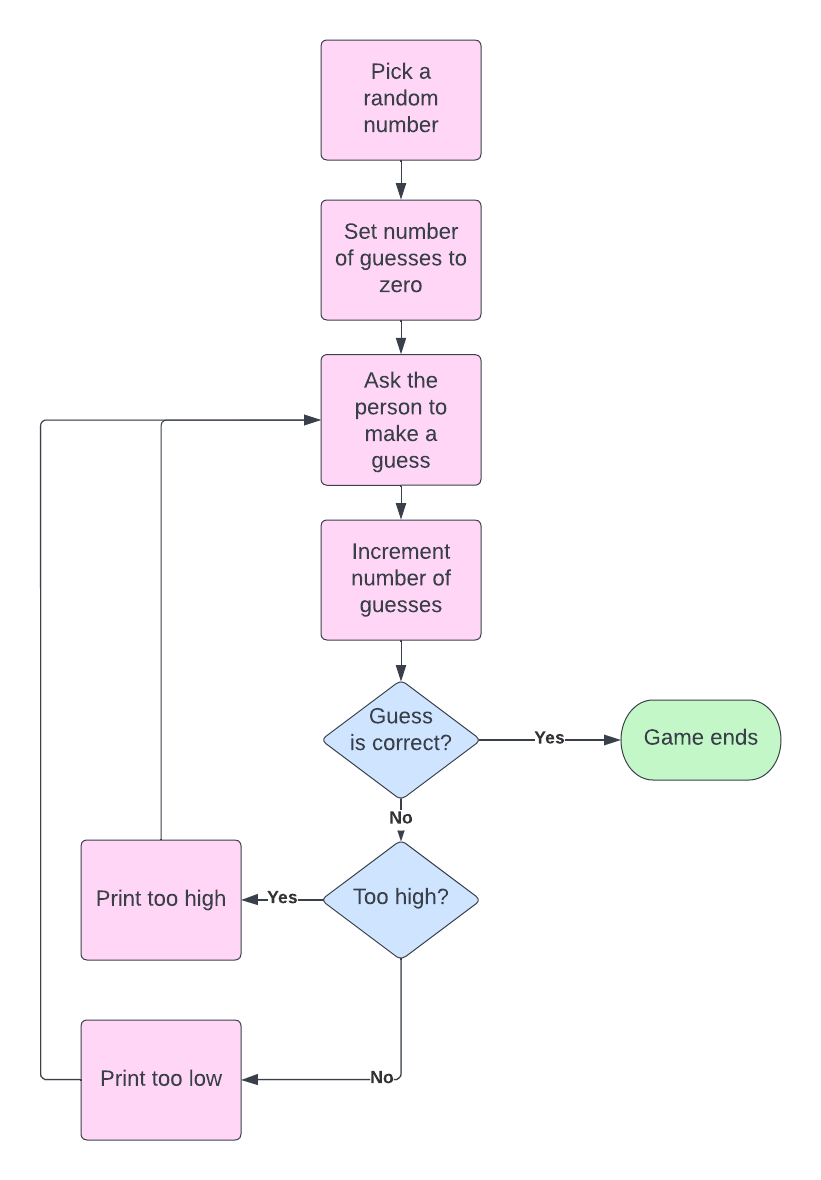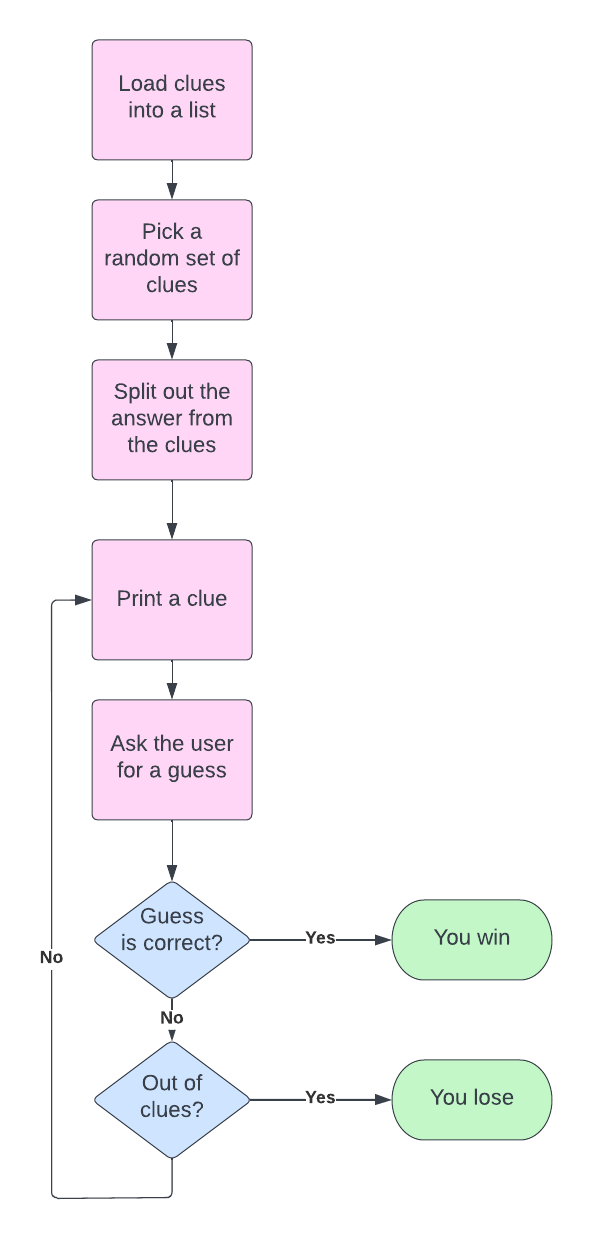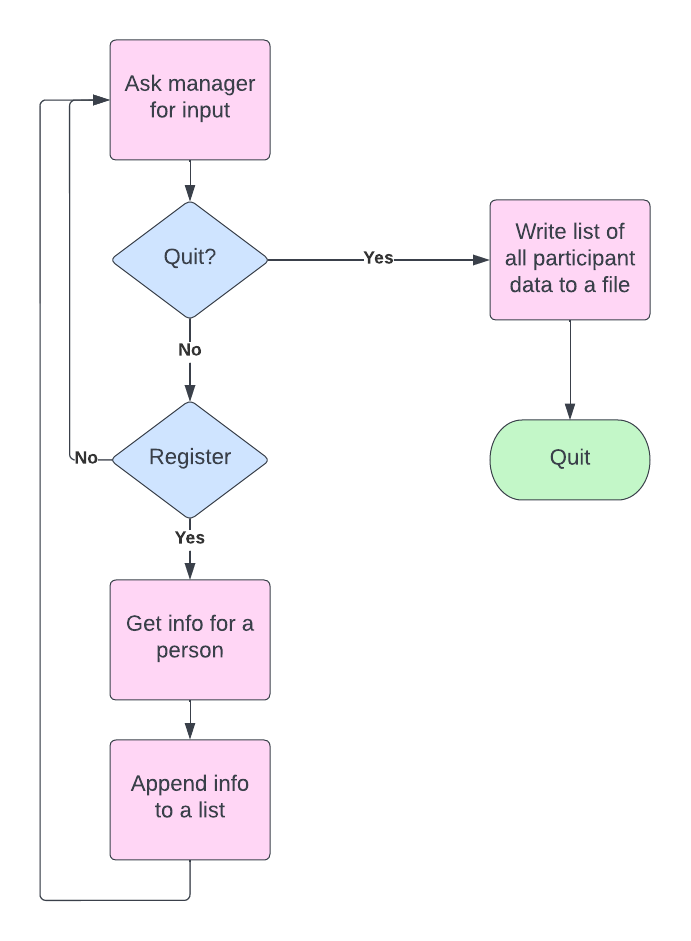Computer Science

# Input

Often you want to get input from the person who is running your program. Maybe you want to ask them to guess a word for a game, or enter their email address and password into a login form.

In Python, you can get input from the keyboard using input(). For example, imagine you are writing a chat bot:

response = input('What is your name: ')
print(f'Hi {response}, how can I help you?')

When you run this code, it will stop and ask you for your name:

What is your name:

After you type a name, such as Emma, then response = 'Emma', and the program will continue. In this case, it will print:

Hi Emma, how can I help you?

## Example: guessing a number

Ask the person to guess a number between 1 and 100. Let them keep guessing until they guess the correct number. Each time they make a guess, if the guess is correct, print “Correct!”, display the number of guesses (‘You took 6 guesses.’), and exit If the guess is lower than the number, print “Lower” If the guess is higher than the number, print “Higher”

We can think of an algorithm to solve this problem using a flow chart:Here is some code to implement this algorithm:

import random

def main():
goal = random.randint(1, 100)
guess_count = 0
while True:
response = input("Guess the number: ")
value = int(response)
guess_count += 1
if value == goal:
print("Correct!")
print(f'You took {guess_count} guesses.')
return
elif value < goal:
print("Higher")
else: # value is > goal
print("Lower")

if __name__ == '__main__':
main()

• We use while True to loop “forever” — until the person running the program enters a correct guess, and then we return from inside the while loop.

• We use random.randint(1,100) to get a random integer between (and including) 1 and 100.

• We use input() to get the guess from the person.

• We convert the guess from a string to an integer — input is always a string until we convert it.

### Notice the if statement at the end

We are using a new if statement at the end — if __name__ == '__main__. This tells Python that if you are running this program normally — python number_guess.py or through PyCharm — then it should run main().

If we are running this program any other way — for example we import it from a test_number_guess.py program, then Python will not run main(). This is really helpful when testing your program or if you are writing a module that someone else is going to import.

## Example — clues

You have a file that contains sets of clues and answers.

Randomly select a set of clues and their associated answer.

Display the clues, one at a time, to the person and prompt a guess. If the guess matches the answer, print “Correct!”, otherwise continue.

If the person runs out of clues, print “I’m sorry, you ran out of clues.” and exit.

### The algorithm

Here is a flow chart that shows an algorithm for this program:### Input file

Before we can write this code, we need to know what the input file looks like. Here is an example:

Nephi
***
A character in the Book of Mormon
He built a boat
His father's name was Lehi
---
Helaman
***
A character in the Book of Mormon
He was named after his dad
He was a chief judge
Kishkumen tried to kill him
---
Jael
***
A character in the Bible
A character in the book of Judges
She was handy with a tent stake
She killed Sisera

Notice that we have the answer first, then clues. The answer is separated from the clues by ***\n. Each block of clues is separated by ---\n.

### The code

Let’s go through the flow chart above and write functions for each piece.

1. Here is a function that can load the clues into a list:
def load_clues(filename):
with open(filename) as file:
return file.read().split('---\n')

When we call file.read(), this reads all of the lines of the file into one big string. We then call split() on this, using ---\n as the delimiter, since this is what separates each block of clues.

1. We can pick a random clue like this:
clue_blocks = load_clues(filename)
clues = random.choice(clue_blocks)
1. We can split out the answer from the clues like this:
def parse_block(clue_block):
clues = clues.strip().split('\n')

answer, clues = parse_block(clue_block)

We use split() with a delimiter of ***\n. We use strip() to take the newline off of the answer. We then split the clues into a list using \n as the delimiter.

1. We can play the game with a function like this:
def play(answer, clues):

for clue in clues:
print(clue)
response = input("Guess: ")
print('Correct!')
return
print("I'm sorry. You ran out of clues.")

This loops through all the cues, prints them one at a time, and lets the player make a guess after each clue is printed.

### Putting it all together

Let’s put all this together:

import random
import sys

with open(filename) as file:

def parse_block(clue_block):
clues = clues.strip().split('\n')

for clue in clues:
print(clue)
response = input("Guess: ")
print('Correct!')
return
print("I'm sorry. You ran out of clues.")

def main(filename):

clue_block = random.choice(clue_blocks)

if __name__ == '__main__':
# main('example_clues.txt')
main(sys.argv)

We use if __name__ == '__main__' at the end again to check if we are running the program normally, and then call main(). Note that if you want to run this program within PyCharm you can comment out the second call to main() and uncomment the first one.

## Example — data entry

You are helping to register people for an event.

Your program should ask whether you want to register a person or quit. The manager types ‘R’ or ‘r’ to register someone, and ‘Q’ or ‘q’ to quit.

If the event manager opts to register someone, they should enter the participant’s first name, last name, and major. Your program will then assign each person randomly to a group (1-6).

When the manager opts to quit, write all the information (name, major, group) for each participant into a file, which will be printed and cut up later to create information slips for each person.

Once the data has been written to the file, print “Information written to {file}”

### The algorithm

Here is a flow chart that shows an algorithm for this program:### The code

Let’s go through this algorithm and write code for each piece.

1. The left hand side is an algorithm to get data for all the users and put it into a list. We could instead get data for one person and write it to the file as soon as we get it. But that would require us sending a file object around in our code. So it is simpler to get all the data at once.

This function gets data for all participants and puts the data into a list:

def get_data():
data = []
while True:
response = input('\nWhat do you want to do?\nRegister a person (R/r)\nQuit(Q/q)\n: ')
if response.lower() == 'q':
return data
elif response.lower() == 'r':
data.append(enter_data())
else:
print(f'Unrecognized option: {response}')

Notice that we are using while True here. This is a common pattern when we need to loop until the person running the program wants to quit. As soon as the manager enters q, then we can return early from this while loop.

As long as the manager enters r we will call enter_data() to get the data for one participant and put it in a list.

1. Here is code to get data for one participant:
import random

def enter_data():
first = input("First name: ")
last = input("Last name: ")
major = input("Major: ")
group = random.randint(1,6)

return first, last, major, group

We need to import random so we can assign a participant to a group. We use random.randint(1,6) to get a random number between 1 and 6 for the group.

We return a tuple from this function! So the get_data() function above is creating a list of tuples. Note that get_data() doesn’t really need to know that it is putting tuples into the list. It just appends whatever enter_data() returns.

1. Here is a function to write all the data to a file:
def write_data(file, data):
for (first, last, major, group) in data:
file.write(f'{last}, {first}\n{major}\nGroup {group}\n\n------------------\n\n')

This iterates over the list of tuples in data and unpacks them. Then it writes to the file, which we assume is open.

### Putting it together

Let’s put all this together with a main() function:

import random

def enter_data():
first = input("First name: ")
last = input("Last name: ")
major = input("Major: ")
group = random.randint(1,6)

return first, last, major, group

def get_data():
data = []
while True:
response = input('\nWhat do you want to do?\nRegister a person (R/r)\nQuit(Q/q)\n: ')
if response.lower() == 'q':
return data
elif response.lower() == 'r':
data.append(enter_data())
else:
print(f'Unrecognized option: {response}')

def write_data(file, data):
for (first, last, major, group) in data:
file.write(f'{last}, {first}\n{major}\nGroup {group}\n\n------------------\n\n')

def main(output_filename):
data = get_data()
with open(output_filename, 'w') as file:
write_data(file, data)
print(f'Information written to {output_filename}')

if __name__ == '__main__':
# main('demo_output.txt')
main(sys.argv)

## An important note

We are showing you quite a few important things here:

• How to use input() to get input from a person running your program.
• How to loop forever using while True and then return when a condition is met.
• Convert input from a string to an integer as needed.
• How to tell if your program is being run or being imported. The __name__ == '__main__' condition is true if your program is being run and false if being imported.
• Returning tuples from functions, storing lists of tuples, and iterating over tuples while unpacking them. Tuples are your friend.
• More practice using random.

Most importantly, we are showing you how to take a problem and develop an algorithm using a flow chart.

If you are having difficulty with developing an algorithm for a problem, don’t despair. It doesn’t mean you are “bad” at programming. You just need more practice!

Try to write a flow chart for every problem you solve. You can get a free education account with https://www.lucidchart.com/pages/usecase/education to quickly make charts.

As you get better at coding, for small problems you can quickly sketch out an algorithm in English or maybe even jump into coding it up. But no matter how much experience you gain over the years, big problems will always require planning and formulating an algorithm. In fact, you will likely have competing algorithms and discussions with colleagues over which algorithm is best. Designing algorithms is one of the most fundamental aspects of programming.